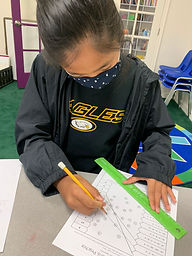## Ms. Kayla

### Target 1​

###### Lesson Type:

Review

Number Operation

:

Number Reltionships

Solve equations to compare their values, recording the results with comparison symbols (>, ≥, ≤, <, and =).

###### 1:

Solve equations using arithmetic properties to compare each equation’s value.

###### 2:

Solve multi-step equations, adhering to arithmetic rules.

6th

###### Vocabulary:

Greater than, Less than, Equal, Expression, Order of Operations, Compare/comparison, Parentheses, Exponent, Multiplication, Division, Addition, Subtraction, Precision, Distributive Property

Activities:

Students used their knowledge of the order of operations to evaluate an expression with up to four operations.

Students compared their solutions to determine which was greater than, less than, or equal to another expression.

Students used the symbols <, >, or = to compare two expressions.### Home Exploration

###### Challenge Problem:

(19 - 8) - 2 is (<, >, =) to 4 + 5(2)

###### Guiding Questions:

Where do you start? What does each symbol mean? Would the answer different if you used the order of operations of distributive property of multiplication?

9 is less than (<) 14## Absent Students:

### Target 2

:

###### 1:

Translate between written sentences and algebraic expressions.

###### 2:

Define an algebraic expression as a combination of numbers, operations, at least one variable, and no equal sign.

6th

###### Vocabulary:

Algebra, Expression, Variable, Translate,

Activities:

Students were given a set of written algebraic expressions in word and numeric form to match.### Home Exploration

Three times a number divided by two

What is the problem describing? What is the given information?

3x/2

###### Guiding Questions:### Target 3

:

###### Vocabulary:

Activities:### Home Exploration